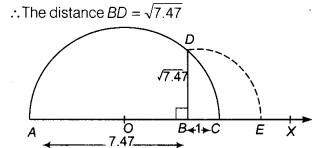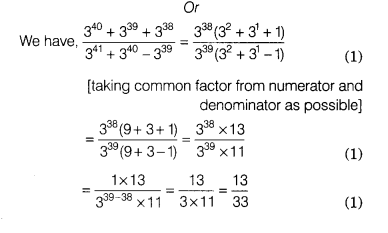# Represent √7.47 on the number line

Represent √7.47 on the number line.

Now, from point S, mark a distance of 1 unit. Let this point be C. Let O be the mid-point of AC.
Now, draw a semi-circle with centre O and radius OA. Let us draw a line perpendicular to AC passing through point B and intersecting the semi-circle at point D.Draw an arc with centre B and radius BD, which intersects the number line at point £, then the point E represents √7.47.